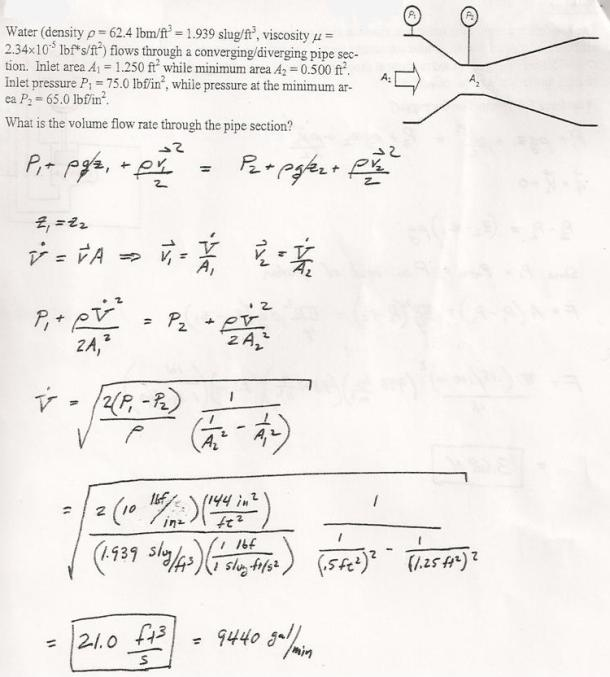fluid mechanics math problems engineering equations fluid mechanics basic mechanics school homework engineering math fluids formulas fluid mechanics problems fluid mechanics problem solutions to fluids problems full solution fluid mechanics engineering fluids problem solution fluid mechanics math problems engineering equations fluid mechanics basic mechanics school homework engineering math fluid mechanics formulas fluid problems fluid mechanics problem solutions to fluid mechanics problems full solution fluid mechanics engineering fluid mechanics problem solution
fluid mechanics math problems engineering equations fluid mechanics basic mechanics school homework engineering math fluids formulas fluid mechanics problems fluid mechanics problem solutions to fluids problems full solution fluid mechanics engineering fluids problem solution fluid mechanics math problems engineering equations fluid mechanics basic mechanics school homework engineering math fluid mechanics formulas fluid problems fluid mechanics problem solutions to fluid mechanics problems full solution fluid mechanics engineering fluid mechanics problem solution
Highalphabet Home Page fluid mechanics solutions fluids math solved Fluid Mechanics Page 1
Water flows through a converging/diverging pipe section. Inlet area A(1)=1.25 ft^2 while minimum area A(2)=.5 ft^2. Inlet pressure P(1)=75 lbf/in^2, while pressure at the minimum area P(2)=65 lbf/in^2. What is the volume flow rate through the pipe section?Water flows through a converging/diverging pipe section. Inlet area A(1)=1.25 ft^2 while minimum area A(2)=.5 ft^2. Inlet pressure P(1)=75 lbf/in^2, while pressure at the minimum area P(2)=65 lbf/in^2. What is the volume flow rate through the pipe section?